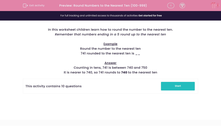# Round Numbers to the Nearest Ten (100-999)

In this worksheet, students round numbers to the nearest ten.This content is premium and exclusive to EdPlace subscribers.Key stage:  KS 2

Curriculum topic:   Maths and Numerical Reasoning

Curriculum subtopic:   Place Value

Difficulty level:#### Worksheet Overview

In this worksheet children learn how to round the number to the nearest ten.

Remember that numbers ending in a 5 round up to the nearest ten

Example

Round the number to the nearest ten

741 rounded to the nearest ten is __